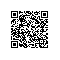# 每日一道算法：删除排序数组中的重复项

## 解法一：双指针法

#### 相关标签

* 数组
* 双指针

#### 思路分析：

1、数组完成排序后，我们可以放置两个指针i和j，其中i是慢指针，而j是快指针。只要nums[i]=nums[j]，我们就增加j以跳过重复项。

2、当我们遇到nums[j]!=nums[i]时，跳过重复项的运行已经结束，因此我们必须把它（nums[j]）的值复制到nums[i+1]。然后递增i，接着我们将再次重复相同的过程，直到j到达数组的末尾为止。

#### PHP代码实现：

/**
* @param Integer[] $nums * @return Integer */ function removeDuplicates(&$nums) {
if(count($nums)==0){ return 0; }$slow = 0;//慢指针
for($fast=1;$fast<count($nums);$fast++){//快指针
if($nums[$slow] != $nums[$fast]){
$slow++;$nums[$slow] =$nums[$fast];//把快指针的值赋值给结束重复项慢指针+1 } } return$slow+1;//数量比指针多1
}
使用：
$nums = [1,2,3,4,5,6,7,8,8,8,9,9,9]; var_dump(removeDuplicates($nums));

#### 复杂度分析：

时间复杂度： O(n)

## 解题关键

1、给定一个排序数组，表示是已经排好序的数组

2、原地修改，即要求空间复杂度为O(1)

3、你不需要考虑数组中超出新长度后面的元素，即表示不用删除重复的元素，可以把重复的元素移动到最后即可

## github

LeetCode_PHP：https://github.com/zhangdejian/LeetCode_PHP

## 题目来源